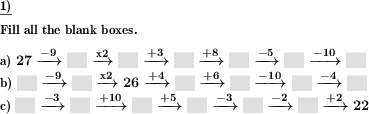Custom math worksheets at your fingertips# Details for problem "Frames & Arrows w/ elementary arithmetic - varying ops"

Quickname: 2247

Elementary School, Primary School, Middle School.

## Summary

In a frames and arrows calculation chain task, the results are to be filled in the blank spaces.

## Example## Description

The task here is to enter the results and intermediate results in a frames and arrows style calculation chain with the operations addition, subtraction, multiplication and division.

The operations to be considered can be selected from:

```- Addition and subtraction
- Addition, subtraction, multiplication and division
```

The arithmetic chain is presented as a series of arrow operators, each carrying one of the arithmetic operations. This operation is to be applied to the previous value, i.e. the initial value or an intermediate result. The result is entered in the box at the right arrowhead and is then considered the first operator of the following task.

In the simplest form, only the starting value is given, the subsequent result boxes are empty and must be filled with the correct numbers according to the task. As a variation of this, only the last value, the final result, can be given. In this case, all operations are to be performed in reverse, i.e. in the form of an inverse operation. A combination of these two settings is the option to specify a number in the middle. Thus, the results in the direction towards the end of the chain have to be calculated according to the arrow labels, the results in the direction towards the beginning of the chain according to the inverse operation of the respective arrow label. In addition, mixed forms of these settings can also be selected.

The number space from which all numbers are taken can be chosen. It can also be determined that negative numbers can occur. The length of the calculation chain and thus the number of calculations to be performed per task can be set.

With regard to the arithmetic symbols for multiplication and division, it can be set whether these are represented as a dot and colon or times symbol and obelus.

Download free printable worksheets for this math problem here. The worksheet contains the problems only, the solution sheet includes the answers. Just click on the respective link.

•Worksheet 1Solution sheet with answers
•Worksheet 2Solution sheet with answers
•Worksheet 3Solution sheet with answers

If you can not see the solution sheets for download, they may be filtered out by an ad blocker that you may have installed. If this is the case, please allow ads for this page and reload the page. The solution sheets will then reappear.

• Do these sample worksheets do not really fit?
• Do you need more math worksheets, with a different level of difficulty?
• Would you like to combine different problems on a worksheet and adjust them to your needs?
• As a teacher, you can put together your own worksheets using the automatically generated math problems provided.
With a free initial credit, you can start creating your own math worksheets in a few minutes.

You can try it for free! Register here, to create custom worksheets now!

## Customization options for this problem

Parameter
Possible values
Number of problems
1, 2, 3, 4, 5, 6, 7, 8, 9, 10
Negative numbers allowed
Yes, No
Results given
First number, Last number, Mid number, First and mid number, Last and mid number, Random
Length of chain
3, 4, 5, 6, 7, 8
Operators
Number range
20, 50, 99, 200, 499, 999, 1999, 4999
Sample problem
Yes, No
Operator notation
· and /, x and ÷, · and ÷, x and /

## Similar problems

Remark
Description
Numbers with or without decimal places have to be added.
Another fill-in problem
Find the next numbers in a series
Simpler form with only addition and subtraction and with the same operation performed in each step
Simple frames and arrows chain task with one elementary arithmetic operation, results are to be filled in blank spaces.
Simple basic division problem
Division of two whole numbers, with remainder, written long division method illustrated.
Another fill-in problem: The magic triangle
In a magic triangle, six numbers have to be filled in correctly.
Simple basic multiplication problems with negative numbers
Multiplication of two negative numbers, training the rules for the product's sign.
Simple basic multiplication problems
Multiplication problems with defined number range
Negative whole numbers are to be added and subtracted
Simple basic subtraction problem
Multiple numbers with or without decimal places have to be subtracted.Deutsche Version dieser Aufgabe
These informational pages with samples describe math problems that can be combined on custom math worksheets with solutions for home and K-12 school use.
Deutsche Seiten
×